# Test: Functions- 3

## 15 Questions MCQ Test Practice Questions for GMAT | Test: Functions- 3

Description
Attempt Test: Functions- 3 | 15 questions in 15 minutes | Mock test for GMAT preparation | Free important questions MCQ to study Practice Questions for GMAT for GMAT Exam | Download free PDF with solutions
QUESTION: 1

### There is water tank already 4/7 full. If Jose adds 5 gallons of water to the water tank, the tank will be 13/14 full. How many gallons of water would the water tank hold if it were full?

Solution:

In this case, we need to solve for the volume of the water tank, so we set the full volume of the water tank as x. According to the question, 4/7 -full  can be replaced as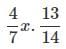-full  would be 13/14x Therefore, we can write out the equation as: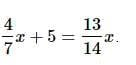Then we can solve the equation and find the answer is 14 gallons.

QUESTION: 2

### There exists a set X = {1, 2, 3, 4}.  Which of the following defines a function of X?

Solution:

Let's look at f,g,and h and see if any of them are functions.

1. f = {(2, 3), (1, 4), (2, 1), (3, 2), (4, 4)}: This cannot be a function of X because two of the ordered pairs, (2, 3) and (2, 1) have the same number (2) as the first coordinate.

2. g = {(3, 1), (4, 2), (1, 1)}: This cannot be a function of X because it contains no ordered pair with first coordinate 2.  Because the set X = {1, 2, 3, 4}, we need an ordered pair of the form (2,  ) .

3. h = {(2, 1), (3, 4), (1, 4), (2, 1), (4, 4)}: This is a function.  Even though two of the ordered pairs have the same number (2) as the first coordinate, h is still a function of X because (2, 1) is simply repeated twice, so the two ordered pairs with first coordinate 2 are equal.

QUESTION: 3

### Let f be a function that assigns x2 to each real number x.  Which of the following is NOT an appropriate way to define f?

Solution:

This is a definition question.  The only choice that does not equal the others is f(y)=x2.  This describes a function that assigns x2 to some number y, instead of assigning x2 to its own square root, x.

QUESTION: 4

If f(x)=x2, find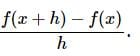Solution:

We are given f(x) and h, so the only missing piece is f(x + h).

f(x+h)=(x+h)2=x2+2xh+h2
Then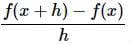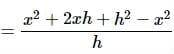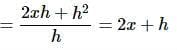QUESTION: 5

Give the range of the function: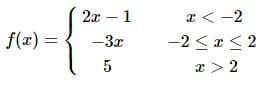Solution:

We look at the range of the function on each of the three parts of the domain. The overall range is the union of these three intervals.

On (−∞,−2), f(x) takes the values:

x<−2
2x−1<2(−2)−1
2x−1<−5
f(x)<−5
or (−∞,−5)

On [−2,2], f(x) takes the values:

−2≤x≤2
−3(−2)≥−3x≥−3(2)
6≥−3x≥−6
−6≤f(x)≤6,
or [−6,6]

On (2,∞), f(x) takes only value 5.
The range of f(x) is therefore (−∞,−5)∪[−6,6]∪{5} , which simplifies to (−∞,6].

QUESTION: 6

A sequence begins as follows:
8,12,...
It is formed the same way that the Fibonacci sequence is formed. What are the next two numbers in the sequence?

Solution:

Each term of the Fibonacci sequence is formed by adding the previous two terms. Therefore, do the same to form this sequence:

8+12=20

12+20=32

QUESTION: 7

Give the inverse of f(x)=5x−7

Solution:

The easiest way to find the inverse of f(x) is to replace f(x) in the definition with y , switch y with x, and solve for y in the new equation.

y=5x−7
x=5y−7
x+7=5y−7+7
x+7=5y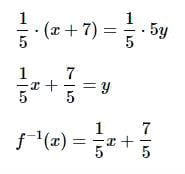QUESTION: 8

Define f(x)=x3−8. Give f−1(x)

Solution:

The easiest way to find the inverse of f(x) is to replace f(x) in the definition with y , switch y with x, and solve for y in the new equation.

y=x3−8

x=y3−8

x+8=y3−8+8

x+8=y3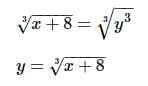QUESTION: 9

Define f(x)=x2−4 and g(x)=x2+4.

Give the definition of (f∘g)(x) .

Solution:

(f∘g)(x)=f(g(x))
=f(x2+4)
=(x2+4)2−4
=x4+8x2+16−4
=x4+8x2+12

QUESTION: 10

Define g(x)=5x−2 .

If g(A)=11, evaluate A.

Solution:

Solve for A in this equation:
g(A)=5A−2=11
5A−2+2=11+2
5A=13
5A÷5=13÷5
A=2.6

QUESTION: 11

Define the operation Θ as follows:

aΘb=ab+100

Solve for x : 4Θx=72

Solution:

4Θx=72
4x+100=72
4x+100−100=72−100
4x=−28
4x÷4=−28÷4
x=−7

QUESTION: 12

Define f(x)=A(x−B)3, where A≠0,B≠0.

Evaluate f−1(1) in terms of A and B.

Solution:

This is equivalent to asking for the value of x for which f(x)=1, so we solve for x in the following equation:
f(x)=1
A(x−B)3=1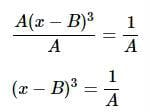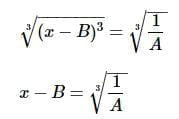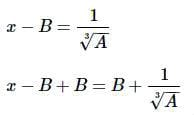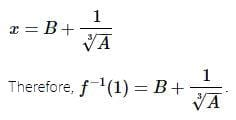QUESTION: 13

Define an operation ⊙ as follows:

For any real numbers a,b ,

a⊙b=(a−1)(b−1)

Evaluate (5⊙5)⊙5.

Solution:

(5⊙5)⊙5
=[(5−1)(5−1)]⊙5
=(4⋅4)⊙5
=16⊙5
=(16−1)(5−1)
=15⋅4=60

QUESTION: 14

An infinite sequence begins as follows:

1,2,−3,4,5,−6,7,8,−9...

Assuming this pattern continues infinitely, what is the sum of the 1000th, 1001st and 1002nd terms?

Solution:

This can be seen as a sequence in which the Nth term is equal to N if N is not divisible by 3, and −N otherwise. Since 1,000 and 1,001 are not multiples of 3, but 1,002 is, the 1000th, 1001st, and 1002nd terms are, respectively,

1000,1001,−1002

and their sum is

1000+1001+(−1002)=999

QUESTION: 15

Define f(x)=√x . What is (f∘f)(x) ?

Solution:

This can best be solved by rewriting √x as1/x2 and using the power of a power property.
(f∘f)(x)=f(f(x)) =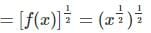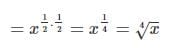Use Code STAYHOME200 and get INR 200 additional OFF Use Coupon Code